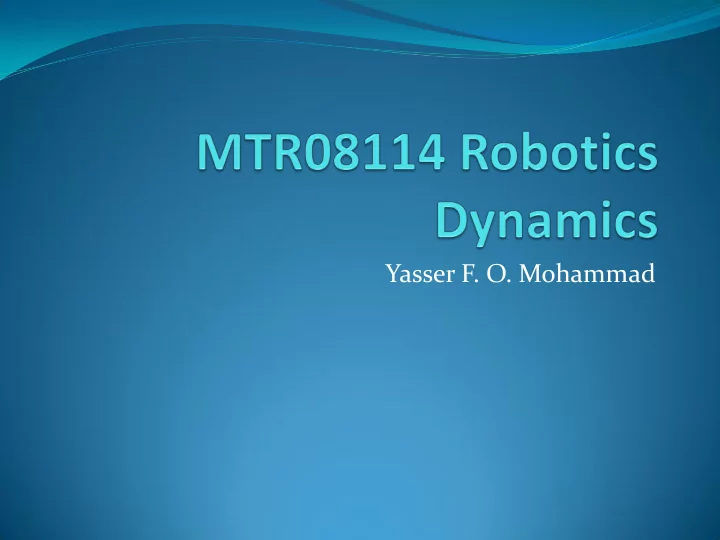# Yasser F. O. Mohammad REMINDER 1: The whole Jacobian (METHOD 1) - PowerPoint PPT Presentation

## Yasser F. O. Mohammad REMINDER 1: The whole Jacobian (METHOD 1) Revolute Joint Prismatic Joint REMINDER 2: Wrist Singularities Whenever z 3 and z 5 are aligned Prove it REMINDER 3: Elbow Singularity REMINDER 4: Resolved Motion

2. REMINDER 1: The whole Jacobian (METHOD 1)  Revolute Joint  Prismatic Joint

3. REMINDER 2: Wrist Singularities  Whenever z 3 and z 5 are aligned  Prove it

4. REMINDER 3: Elbow Singularity

5. REMINDER 4: Resolved Motion Rate Control (Whitney 1972) From Osama Khatib

6. REMINDER 5: How to calculate J +  Most difficult method (from definition):      1 T T J J J J  Simplest Method (SVD):     T J U V     1/ , when 0 ij ij ij     T J U V

7. REMINDER 6: Manipulability m     ii  i 1 If the robot is not redundant (n<=6)   det J

8. REMINDER 7: Velocity Force Duality    J   T J F   f    F   n

9. REMINDER 8: How to do the elimination From Osama Khatib

10. What is dynamics  Relationship between forces and motion  Two approaches:  Traditional Newton laws approach  Eular-Lagrange formulation  In robotics we use Eular-Lagrange formulation because it is MUCH easier for complex objects.

11. Motivation (1D system)

12. Eular- Lagrange Equation

13. Kinetic Energy Inertia Tensor Translation Rotation

14. Calculating Inertia Tensor in Fixed Frame

15. Example (Uniform Solid)

16. Moving Inertia Tensor Between Frames

17. Kinetic Energy for N Links Robot

18. Potential Energy for N Link Robot

19. Eular-Lagrange Equation of a Robot Christoffel Symbols of the first order

20. Example 2D Cartesian (PP)

21. Planar Elbow

22. Planar Elbow

23. Planar Elbow

24. Planar Elbow

More recommend